Course Content

0/4

0/3

0/1

0/2

0/1

0/4

0/3

0/5

0/3

0/5

0/5

0/1

0/6

0/2

0/4

0/5

2.2 The Discriminant of Quadratic Equations 一元二次方程式的根的判别式



The quadratic equation kx+ 2(k + a)x + (k + b) = 0 has equal roots. Express k in terms of a and b.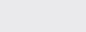Find the range of k where the equation kx+ x + 2 = (x + 1)has two unequal real roots.

k < 1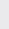The quadratic equation (p + 1)x+ 2px + (p + 2) = 0 has real roots. Find the range of values of p.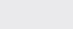Prove that 2ax+ (3a + 2c)x + 2c = 0 has distinct real root for all real value of x.



Find the value of k so that the line kx + y = 8 is the tangent of the curve x+ xy = 4.

5


Prove that, for all real value of a, the equation x+ 2ax + 2a+ a + 1 = 0 has no real roots for x.



7Show that the line x + y = q will intersect the curve x– 2x + 2y= 3 in two distinct point if q< 2q + 5. 试证若q< 2q + 5，直线x + y = q 与曲线x– 2x + 2y= 3 交于两个相异的点。



Find the range of the values of c for which 3x+ 5x +c is always positive.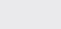If the quadratic function f (x) = (a – 2)x– 2ax + a + 1 is never positive, find the greatest value of a.

-2


Find the values of a for which ax+ 3x + 4a is

(a) positive for all the real values of x,

(b) negative for all the real values of x.

(a) a >(b) a <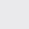Prove that (mx – 1)(x – 2) = m has two distinct real roots for xR.